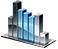# Arrays

Arrays are designed to hold a series of elements of the same type.

There are two types of arrays in the NetTradeX language:

• Dynamic arrays
• Arrays with reverse indexing

## Dynamic arrays

The size of a dynamic array can be variable, which means the volume of memory is allocated for the array and determined while the program is running.

Indexing of elements starts from 0, so the first element is at 0 index, the second is at 1, etc.

Before using an array, it must be declared. Declaration of an array includes:

• key word `array`
• data type, kept in the array, enclosed in angle brackets`<>`
• array name

Example:

array <int> arr;

To initialize elements of the array arr while declaring it, the following syntax should be used:

array <type> name = {element1,element2,...};

The array of N elements of int type can be created as follows:

array <int> name(N);

To simultaneously create an array and initialize all the elements of the same value, the following construction should be used:

array <type> name(number of_elements, value);

The number of elements in the created array can be calculated using `length()` method by calling array_name.length().

To access the array elements, use the operator `[]`. The number of an element to be accessed is placed within the square brackets.

Arrays starts from zero, so if there is an array `arr` and there is a need to access its fifth element, you should use `arr`.

Example:

` int Initialize() {return(0);} int Run() { array <int> arr = {10,20,30,40,50}; for(uint i=0;i<arr.length();i++) { System.Print("arr["+i+"]="+arr[i]); } return(0); } int DeInitialize(){return(0);} `

Output:

` arr=10 arr=20 arr=30 arr=40 arr=50 `

Two-dimensional arrays can be created in the following ways:

` array<array<int>> a; array<array<int>> b = {{1,2},{3,4}}; array<array<int>> c(10, array<int>(10)); `

Accessing elements of such arrays will be the following: `array_name[index1][index2]`

Let us demonstrate how two-dimensional arrays work with an example of the matrix transposition:

` int Initialize(){retuindexingrn(0);} array<array<int>> transpose(array<array<int>> alfa) { int newLines = alfa.length(); int newRows = alfa.length(); array<array<int>> beta(newLines,array<int>(newRows)); for (int i=0;i<newRows;i++) { for(int j=0;j<newLines;j++) { beta[j][i]=alfa[i][j]; } } return beta; } void Array2DPrint(array<array<int>> alfa) { string data=""; for(uint i=0;i<alfa.length();i++) { for(uint j=0; j<alfa[i].length(); j++) { data +=" ["+i+"]["+j+"]="+alfa[i][j]; } } System.Print(data); } int Run() { array<array<int>> b = {{1,2},{3,4},{5,6}}; System.Print("Initial matrix"); Array2DPrint(b); System.Print("Transposed matrix"); Array2DPrint(transpose(b)); return 0; } int DeInitialize(){return(0);} `

Output:

` Initial matrix =1 =2 =3 =4 =5 =6 Transposed matrix =1 =3 =5 =2 =4 =6 `

Arrays may be used with operation ` = ` for assignment and ` == ` / `! = ` for checking equality/inequality of all array elements.

Example:

` int Initialize() { return(0); } int Run() { array <int> a={10,11,12,13}; array <int> b; b=a; for(uint i=0;i<b.length;i++) { System.Print("b["+i+"]="+b[i]); } System.Print("Are the arrays equal? "+(a==b)); b=1; System.Print("Are the arrays equal? "+(a==b)); return(0); } int DeInitialize() { return(0); } `

Each dynamic array has a number of methods for operating its elements. These methods are listed below:

• `uint length() const` - the number of elements in an array
• `void resize(uint)` - changing the length of the array
• `void reverse()` - inverting the order of elements
• `void insertAt(uint index, const T& in)` - inserting an element`in` in the position `index`
• `void insertLast(const T& in)` - inserting an element`in` at the end of the array
• `void removeAt(uint index)` - deleting an element at the position `index`
• `void removeLast()` - deleting the last element
• `void sortAsc()` - sorting in the ascending order
• `void sortAsc(uint index, uint count)` - sorting in the ascending order`count` of elements starting from the position `index`
• `void sortDesc()` - sorting in descending order
• `void sortDesc(uint index, uint count)` - sorting in descending order`count` of elements starting from the position `index`
• `int find(const T& in)` - search for the element `in` in the array
• `int find(uint index, const T& in)` - search for the element `in`, starting from the position `index`

Example of operating arrays methods:

` int Initialize(){return(0);} string array_print(array <int> data) { string temp =""; for(uint i=0;i <data.length; i++) { temp += data[i]+" "; } return temp; } int Run() { array<int>data={4, 1, 7, -1, 2, 3, -7, 5, -12}; System.Print("Initial array: " + array_print(data)); // Displayed Initial array: 4 1 7 -1 2 3 -7 5 -12 System.Print("length="+data.length()); // Displayed length=9 data.resize(7); System.Print("resize="+ array_print(data)); // Displayed resize=4 1 7 -1 2 3 -7 data.reverse(); System.Print("After reverse: " + array_print(data)); // Displayed After reverse: -7 3 2 -1 7 1 4 data.insertAt(2,9); System.Print("After insert: " + array_print(data)); // Displayed After insert: -7 3 9 2 -1 7 1 4 data.removeAt(3); System.Print("After remove: " + array_print(data)); // Displayed After remove: -7 3 9 -1 7 1 4 data.sortAsc(1,3); System.Print("After sort ascending: " + array_print(data)); //Displayed After sort ascending: -7 -1 3 9 7 1 4 data.sortDesc(1,4); System.Print("After sort descending: " + array_print(data)); //Displayed After sort descending: -7 9 7 3 -1 1 4 System.Print("Position of 7 is: " + data.find(7)); // Position of 7 is: 2 return 0; } int DeInitialize(){return(0);} `

## Arrays with reverse indexing

Arrays with reverse indexing are mainly designed to store the buffer indicator values and used as one of the parameters of the function SetIndexBuffer. To create an array with the reverse indexing the following syntax should be used:

type array_name [dimension];

The dimension determines the number of array elements. The array elements may be accessed using the square brackets `[]`, specified after the array name.

To create a zero-length array the following syntax should be used: type array_name[]; or type[] array_name;

Indexing of elements starts from the end of an array, which means the element at index 0 - the last, at index 1 - the penultimate, etc.

Only non-negative integers are allowed as an index.

Example:

` int Initialize() {return(0);} int Run() { int a; a=11; a=22; a=33; System.Print("a="+a); return(0); } int DeInitialize(){return(0);} `

Output:

a=22